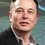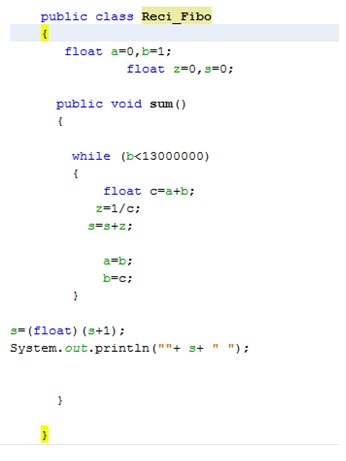# Play with Fibonacci

The $\text{Fibonacci Sequence}$ is a sequence of integers where the first $\displaystyle 2$ term are $\displaystyle 0$ and $\displaystyle 1$, and each subsequent term is the sum of the previous two numbers.

$0 , 1 , 1 , 2 , 3 , 5 , 8 , 13 , 21 , 34 , 55 , 89 , 144 , 233 , 377, ...$

The $n$-th Fibonacci number is denoted by $F_n$ and the sequence is defined by the recurrence relation

$\large F_n = F_{n-1} + F_{n-2}.$

Here, $F_0 = 0,\enspace F_1 = 1,\enspace F_2 = F_1 + F_0 = 1$ etc. 

$\large F_n = \dfrac{ \left( \dfrac{1+\sqrt{5}}{2} \right)^{n}- \left(\dfrac{1-\sqrt{5}}{2} \right)^{n} }{\sqrt{5}}$

$\text{Problem 1:}~$(By - Jake Lai) Prove that for all - $\large |x| < \phi^{-1}$

$\large \displaystyle \dfrac{x}{1-x-x^{2}} = \sum_{k=0}^{\infty} F_{k}x^{k}$

$\text{Problem 2:} ~~ \large \displaystyle \sum_{i=0}^{n} \dfrac{F_{i}}{i}$

$\text{Problem 3:} ~~ \large \displaystyle \sum_{i=1}^{n} \frac{1}{F_{i+1}\times F_{i}}$

$\text{Problem 4:} ~~\large \displaystyle \sum_{i=1}^{n} \frac{1}{F_{i}}$

$\text{Problem 5:}$ By Azhaghu Roopesh M

$\large \displaystyle \sum_{p=0}^{n-1} \binom{n-p}{p} = F_{n+1}$Note by U Z
6 years, 5 months ago

This discussion board is a place to discuss our Daily Challenges and the math and science related to those challenges. Explanations are more than just a solution — they should explain the steps and thinking strategies that you used to obtain the solution. Comments should further the discussion of math and science.

When posting on Brilliant:

• Use the emojis to react to an explanation, whether you're congratulating a job well done , or just really confused .
• Ask specific questions about the challenge or the steps in somebody's explanation. Well-posed questions can add a lot to the discussion, but posting "I don't understand!" doesn't help anyone.
• Try to contribute something new to the discussion, whether it is an extension, generalization or other idea related to the challenge.

MarkdownAppears as
*italics* or _italics_ italics
**bold** or __bold__ bold
- bulleted- list
• bulleted
• list
1. numbered2. list
1. numbered
2. list
Note: you must add a full line of space before and after lists for them to show up correctly
paragraph 1paragraph 2

paragraph 1

paragraph 2

[example link](https://brilliant.org)example link
> This is a quote
This is a quote
    # I indented these lines
# 4 spaces, and now they show
# up as a code block.

print "hello world"
# I indented these lines
# 4 spaces, and now they show
# up as a code block.

print "hello world"
MathAppears as
Remember to wrap math in $$ ... $$ or $ ... $ to ensure proper formatting.
2 \times 3 $2 \times 3$
2^{34} $2^{34}$
a_{i-1} $a_{i-1}$
\frac{2}{3} $\frac{2}{3}$
\sqrt{2} $\sqrt{2}$
\sum_{i=1}^3 $\sum_{i=1}^3$
\sin \theta $\sin \theta$
\boxed{123} $\boxed{123}$

Sort by:

Problem 4:

Given below is a Java Code (Netbeans IDE)Sorry, I couldn't get the code arranged properly using LaTex as @Brock Brown does, so I had to upload a pic .

@megh choksi , I don't think an accurate partial sum can be developed, so I just let the upper bound be a very large number since I guessed that it will converge(yes it does !) .

Can you write a Mathematical solution ? Take the $Lim_{n \rightarrow \infty}$

- 6 years, 5 months ago

@megh choksi Also remember $F_0 = 0$ so change the lower bounds of the summations wherever necessary :)

- 6 years, 5 months ago

Thanks edited

- 6 years, 5 months ago